## Monday, December 30, 2013

### Predictive Analytics in Tableau Part 1: Simple Linear Regression

Today, we will begin the next series of posts about performing predictive analysis via Tableau 8.1's new R functionality.  More specifically, we'll be talking about Simple Linear Regression.  Some of you may remember our previous post on this topic, Performing Simple Linear Regression in Tableau.  That procedure utilized Table Calculations, which despite being powerful in their own right, were not quite meant for such complex mathematics.  The new R integration makes this task significantly easier, as we are about to see.  For this procedure, we will use a sample data set from a collegiate source.

The first question you might ask is "Why is this important?"  Well, regression is one of the many ways in which you can predict new observations.  Want to know what your sales will be next month for a particular product line?  Regression can help.  Want to know how many new customers you will acquire in the next 3 months?  Regression can help.  The list goes on and on.  Now, all we need is a good foundation.  Then, addressing many of your business problems would be within our grasp.

The first step of any regression model is determining which variables are going to be your predictors and which variables are going to be your responses.  In a simple linear regression model, we can only have one predictor and one response.  We also assume that they are related in a "linear" fashion, which is easiest understand via a picture.Linear vs. Nonlinear
Now, we can see that the relationship between Foreign and Consumer is approximately linear.  However, some of you might ask, "What are these values?"  These are U.S. Economic figures for the years between 1976 and 1987.  Foreign is "Foreign Investments / Billions of Dollars" and Consumer is "Consumer Debt / Billions of Dollars."  Now, let's try to predict how much we will have in foreign investments given that we know what consumer debt will be.Foreign (Predicted)
The code for creating a linear regression model is extremely simple, nothing more than two lines of actual code.  Now, let's see what values we get.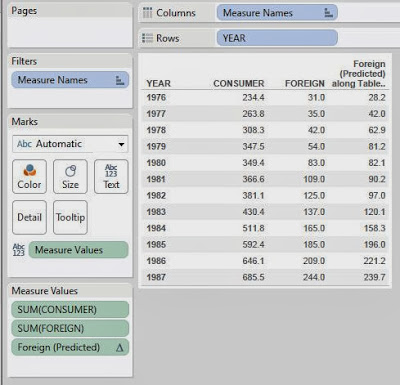Consumer, Foreign, and Predicted Foreign by Year
Voila!  We have predictions.  However, it's difficult to see the relationship in a text table.  Let's create a % Difference calculation and make this into a highlight table.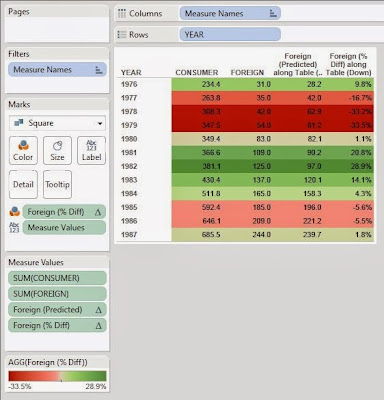Consumer vs. Foreign (Highlight Table)
Now we can easily see which predictions were close, and which were not so close.  This was a bit too easy though, let's try something else.  When you use a regression model to predict a value, you don't just get a single value, you actually get a range that the value is likely to be in.  This is called a prediction interval.  If you want a more rigorous definition, you can check out this article.  Now, what if we were to use R to calculate these intervals?  Let's see!Foreign (Predicted Lower)Foreign (Predicted Upper)
These calculations leverage a neat function called predict() that we won't go into detail on at this time.  However, it's one of R's many multi-purpose functions.  Now, we just need a way to look at these.  A little imagination goes a long way in these types of charts.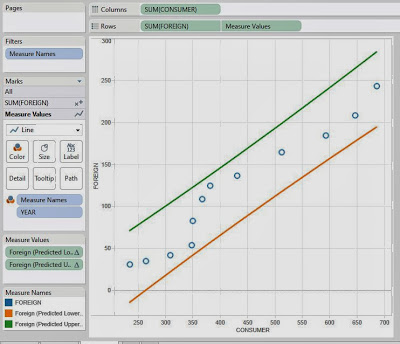Consumer vs. Foreign (Banded Scatterplot)
As you can see, all of our values fall within the bounds.  This is a good thing.  It means that our model fit our data pretty well.

The really cool part about this new R integration is definitely in the prediction and forecasting scenarios.  As we just saw, it's really easy to get some cool predictions and display them.  There's WAY more to do here.  We could have gotten more technical by looking at residual plots or QQ plots.  Don't think that linear regression was the right model?  No problem!  We could have used a time series, artificial neural network, or even a Bayesian model.  You're only limited by your imagination.  We hope you found this informative.  Thanks for reading.

Data Analytics Consultant
Mariner, LLC
http://breaking-bi.blogspot.com

## Monday, December 16, 2013

### Tableau vs. Power Pivot Part 13: Many-to-Many Relationships

Today, we will talk about resolving slightly more complex many-to-many (M2M) relationships.  In case you missed our introductory post on this topic, you can find it here.  In this example, we have a set of sales orders.  Also, each order can be assign to one or more sales reasons.  This also means that each sales reason can be assigned to one or more sales orders.  The data set we are using comes from the AdventureWorks database.

First, let's look at these tables.Many-to-Many Relationship
You  might ask, "Why can't we just join these tables together since we have keys?"  We'll show you why.Total Due for 2006 with Sales Reason
This is the Total Due for all Orders in 2006.  Now, let's see what happens if we join the tables together.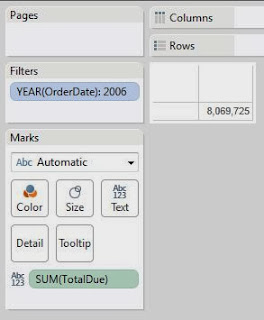Joined Total Due for 2006 with Sales Reason
As you can see, this total is much higher than the actual total.  Why?  When you join across a M2M, you introduce duplicates into your data set.  Now, on to the business question.  We want to know how much money is generated from each Sales Reason for each Month in 2006.  Let's see how Power Pivot would solve this.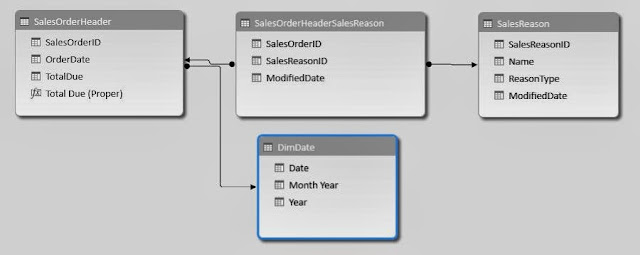Many-to-Many Relationship (Power Pivot)
In order to report on the Month, we needed to add a Date dimension.  The rest of the model remains the same.  Now, let's make our pivot table.Total Due for 2006 by Month and Sales Reason (Power Pivot)
As you can see, the same value is replicated for each column.  The totals are way too high as well.  This is what happens when you try to query across a M2M.  Now, let's fix it.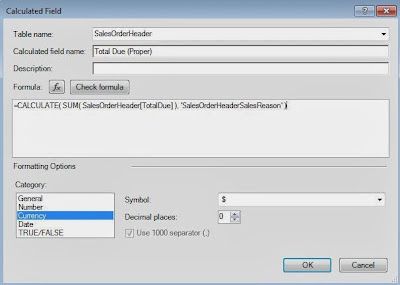Total Due (Proper) (Power Pivot)
Remember this formula from Tableau vs. Power Pivot Part 12: Introductory Data Modeling?  This is the magic formula that allows Power Pivot to query across a M2M.  Let's see the results.Total Due for 2006 by Month and Sales Reason (Proper) (Power Pivot)
The grand total now equals what it was supposed to.  If we look at the totals for the first 6 months, we see the issue.  The Manufacturer and Quality columns were complete duplicates.  However, it doesn't matter because Power Pivot realized this and calculated the totals accordingly.  Now, let's see how Tableau deals with this.Simple Many-to-Many Blend (Tableau)
As we saw in our previous post on this topic, blending can take care of simple M2M relationships.  Namely, if you are reporting on the same fields you are blending on, then the totals add up perfectly.  However, when you try to report on columns that you aren't blending on, you run into problems.Many-to-Many Blend with Extra Dimension (Tableau)
As you can see, adding the Name field doesn't change the granularity, yet still breaks the totals.  This will be a major problem if we try to blend in this scenario.  For kicks, here's what it would look like.Total Due for 2006 by Month and Sales Reason (Blend) (Tableau)
There are so many things wrong with this chart, we won't even talk about it.  Let's move on to the proposed solution.  Since we can't blend in the dimensions, we will need to join them in.Total Due for 2006 by Month and Sales Reason (Naive Join) (Tableau)
The chart looks great, except that the totals are wrong.  In fact, we showed that this would happen already.  However, now that we have the dimensions, can we alter the measures to remove the duplicates?  To see this, let's break this chart up into 4 areas.Chart Areas (Tableau)
We will refer to these areas by the following names: Red = Primary, Orange = Month Totals, Green = Name Totals, Blue = Grand Total.  Now, we can see that the Red and Green areas sum up correctly.  However, the Orange and Blue Areas are being inflated due to the duplicates.  So, the first step is to identify the duplicates.Total Due and Rank by Sales Order and Sales Reason (Tableau)
Using a ranking method, we can see that every value with an Index greater than 1 is a duplicate.  However, we did this with a table calculation which would cause a mess of other issues.  So, we need to push this ranking method directly into the data source.  We can do this by adding a single piece to Custom SQL SELECT statement.

RANK() OVER (
) AS Duplicate

This piece of code will give us the same values we saw using the Index, without using a table calculation.Total Due for 2006 by Name and Month (Filtered Join) (Tableau)
By filtering out the duplicates, we were able to fix our totals.  However, doing this also removed those values from the Primary and Name Total areas, which is not what we wanted.  We want the duplicates in the Primary and Name Total areas, but don't want them in the Month Total and Grand Total areas.  Remember that each of these names also corresponds to an ID number?  We can use that to our advantage.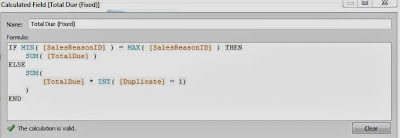Total Due (Fixed) (Tableau)
Each value in the Primary and Name Total areas corresponds to a single Sales Reason Name.  Therefore, they must also correspond to a single Sales Reason ID.  Also, when you only have one value, then MIN() = MAX().  So, the first section of the IF statement only affects the Primary and Name Totals areas, while the second section of the IF statement affects the Month Totals and Grand Total areas.  Now, when we are in the Primary and Name Totals areas, we don't want to change our values at all.  However, when we are in the Month Totals and Grand Total area, we want to remove the duplicate.  The [Duplicate] = 1 statement returns True/False.  When you wrap a True/False value in the INT() function, you get a 1/0 result, respectively.  Therefore, we can remove the duplicate values by multiplying by this 1/0 result.  Now, let's see if this works.Total Due for 2006 by Month and Name (Fixed) (Tableau)
As you can see, we have fixed our calculation.  However, this took a significant amount of work and knowledge, as well as a good amount of ingenuity.  Comparing this to Power Pivot, which was a 1 line measure, it was an easy decision.

Winner: Power Pivot

Thanks for reading.  We hope you found this informative.

Data Analytics Consultant
Mariner, LLC
http://breaking-bi.blogspot.com

## Monday, December 9, 2013

### Performing K-Means Clustering in Tableau

Today, we will talk about performing K-Means Clustering in Tableau.  In layman's terms, K-Means clustering attempts to group your data based on how close they are to each other.  If you want a rudimentary idea of how it looks, check out this picture.  Avid readers of this blog will notice that some of our previous posts, such as Simple Linear Regression and Z Tests, attempted to bring some hardcore statistical analysis into Tableau.  These posts required an extensive knowledge of statistics and Tableau.  Now, with Tableau 8.1's R integration, we can do even cooler stuff than that.  For those of you that don't know, R is a free statistical software package that is heavily used by Academic and Industry statisticians.  The inspiration for this post came from a post on the Tableau website.  You can read it here.

Microsoft's "Data Mining with SQL Server 2008" gave a perfect analogy for why you would want to use a clustering algorithm.  Feel free to read it here.  With this in mind, imagine that you have a bunch of demographic data about your customers, age, income, number of cars, etc.  Now, you want to find out what groups of customers you really have.  Take a look at some sample data we mocked up.Sample Data
First, we need to examine our attributes.  In order to do this, we need to understand the difference between discrete and continuous attributes.  Simply put, a discrete attribute has a natural ordering, yet can only take a small number of distinct values.  Number of Children is a great example of this.  The values are numeric, which gives them a distinct ordering and there's a natural cap to how high this number can be.  It's extremely uncommon to see anyone with more than six children or so.  On the other hand, a continuous attribute can still be ordered, but takes far too many values for you to be able to list them all.  Income is a great example of this.  If you lined up all of your friends in a room, it's extremely unlikely that any of you would make the same exact amount of money.  An easy way to distinguish between these is to ask yourself, "Could I make a pie chart out of this attribute?"  If you answered yes, then the attribute is discrete.  If you answered no, then it is continuous.  Please note that this a gross oversimplification of these definitions, but they are good enough for this post.  Feel free to google them if you want to know more.

Now, let's see kinds of attributes we have.

Customer ID:           Unique Key
Age:                        Continuous
Education:                Discrete
Gender:                    Discrete*
Number of Cars:      Discrete
Number of Children: Discrete
Yearly Income:         Continuous

*Gender is technically a categorical attribute.  We'll touch back on this later.

Another important thing to note is that the K-Means Algorithm in R requires numeric input.  Therefore, we had to replace Education and Gender with numeric IDs.  Partial High School is a 1 and Doctorate Degree is a 6, with everything in the middle ordered appropriately.  Female is 0 and Male is 1.  This would have been an issue if we had a categorical attribute with more than two levels.

Now, we should also note that the R scripting functions qualify as Table Calculations.  Therefore, you need to set up your canvas before you can send the appropriate values to R.  Let's start by setting Customer ID on the Detail Shelf.  This defines the granularity of the chart.Customer ID
Now, we need to create the calculated field that will create the clusters.  This code is commented (comments in R start with #) so that you can read it more easily.

SCRIPT_INT("
## Sets the seed

set.seed( .arg8 )

## Studentizes the variables

age <- ( .arg1 - mean(.arg1) ) / sd(.arg1)
edu <- ( .arg2 - mean(.arg2) ) / sd(.arg2)
gen <- ( .arg3 - mean(.arg3) ) / sd(.arg3)
car <- ( .arg4 - mean(.arg4) ) / sd(.arg4)
chi <- ( .arg5 - mean(.arg5) ) / sd(.arg5)
inc <- ( .arg6 - mean(.arg6) ) / sd(.arg6)
dat <- cbind(age, edu, gen, car, chi, inc)

num <- .arg7

## Creates the clusters

kmeans(dat, num)\$cluster
",

MAX( [Age] ), MAX( [Education ID] ), MAX( [Gender ID] ),
MAX( [Number of Cars] ), MAX( [Number of Children] ), MAX( [Yearly Income] ),
[Number of Clusters], [Seed]
)

Basically, this code sends our six attributes to R and performs the clustering.  We also passed two parameters into this code.  First, we made a parameter that can change the number of clusters.  Second, we made a parameter that sets the seed.  A seed is what determines the output from a "random" number generator.  Therefore, if we set a constant seed, then we won't get different clusters every time we run this.  This is EXTREMELY important to what we are about to do.

Now, we want to examine our clusters on an attribute-by-attribute basis so that we can determine what our clusters represent. In order to do this, we made the following chart: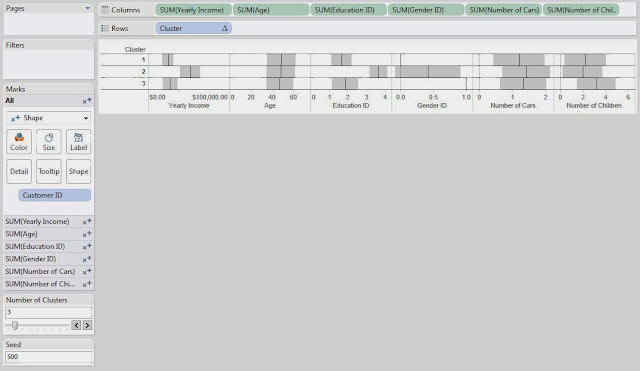Clusters (Seed 500)
This chart is nothing more than a bunch of Shape charts, with the Transparency set to 0 so that we can't see the shapes.  Then, we put -1,+1 standard deviation shading references and an average reference line on each chart.  Next, our goal is to look at each chart to see how the clusters differ.  We will use very rough estimation when we look at these charts.  Don't beat yourself up over the tiny details of which box is bigger or which line is higher; this isn't an exact science.

On the Yearly Income chart, we see that Clusters 1 and 3 are pretty close, and Cluster 2 is much higher.  So, we'll say that Cluster 2 is "Wealthy."

On the Age chart, we don't see a significant difference between any of the Clusters.  So, we move on.

On the Education ID chart, we see that Clusters 1 and 3 are pretty close again, and Cluster 2 is much higher.  So, we'll call Cluster 2 "Educated."  Shocking Surprise!  Educated People seem to make more money.  It's almost like we built the data to look like this.  Anyway, moving on.

On the Gender ID chart, we see that Cluster 1 is almost entirely female and Cluster 3 is almost entirely male.

On the Number of Cars chart, we don't see a significant difference between the clusters.

On the Number of Children chart, we see that Cluster 3 has more children than the other clusters.  So, we'll call this Cluster "Lots of Children".

Now, let's recap our clustering:

Cluster 1: "Male."  We'll call this cluster the "Average Males"
Cluster 2: "Wealthy", "Educated."  We'll call this cluster "Wealthy and Educated"
Cluster 3: "Female", "Lots of Children."  We'll call this cluster "Females with Lots of Children"

Now, before anybody cries sexism at us, we will say that we intentionally created a relationship between income and education.  However, the fact that gender and number of children were clustered together were purely random chance.

This leads us to another question.  What if you don't like the clusters you got?  What if they weren't very distinguishable or you thought that the random number generator messed up the clusters.  Easy!  Just change the seed and/or the number of clusters.Clusters (Seed 1000)
Changing the seed to 1000 completely changed our clusters.  This is what's so cool about statistics.  There are no right answers.  Everything is up for interpretation.

Now, you might ask, "If the clusters change every time, why is this even useful?"  That's the singular defining question behind statistics and the answer is typically, "Run it more than once."  If you create 10 sets of clusters and 9 of them pair High Income with High Education, then that's a VERY good indication that your data contains that cluster.  However, if you run it 10 times and find that half of the time it groups Men with High Income and the other half of the time it groups Women with High Income, then that probably means there is not a very strong relationship between Gender and Income.

We're sorry that we couldn't explain in more depth how the R functionality or the clustering algorithm works.  It would probably take an entire book to fully explain it.  Feel free to do some independent research on how clustering algorithms work and how to interpret the results.  We encourage you to experiment with this awesome new feature if you get a chance.  If you find a different, and maybe even better, way of doing this, let us know.  We hope you found this informative.  Thanks for reading.

P.S.

We would have much rather represented our discrete attributes, or categorical in the case of Gender, using some type of bar graph.  However, we were completely unable to find a way to get it to work.  If you know of a way, please let us know in the comments.

Associate Data Analytics Consultant
Mariner, LLC
http://breaking-bi.blogspot.com

## Monday, December 2, 2013

### Tableau vs. Power Pivot Part 12: Introductory Data Modeling

Today, we will talk about Introductory Data Modeling.  These models will be slightly more advanced that the models we saw in Tableau vs. Power Pivot Part 3: Basic Data Modeling.  However, these are all tasks that a business analyst would face in the real world.  For this examination, step into the shoes of an analyst working at a bank.  We will use two mock-up data sets during this analysis.

Model 1: Fact Tables of Different Granularity

We have a list of transactions per customer as well as a list of closing balances for those customers.  Let's take a look at these data sets.TransactionsClosing Balances (Multiple Fact Tables)
Now, your job is to make sure that these numbers add up.  Therefore, you need a way to combine the fact tables to do your analysis.  Let's see how Power Pivot would handle this.Multiple Fact Tables with Different Granularities (Power Pivot)
It is important to note that every dimension we want to slice by needs its own table in the model.  It is also important to note that we created a Month Year dimension.  This is an extremely important dimension and the model would fail without it.Closing Balance by Account and Month Year (Power Pivot)
Closing Balance was extremely easy because the fact table already existed at this granularity.  We simply used the pivot table to display the data we already had.  However, getting the totals and subtotals to calculate correctly is a much more complex task that will be handled in a later post.  For now, we simply chose to hide them.  Now, we need to create a calculate that adds up our transactions in order to compare them to the closing balances.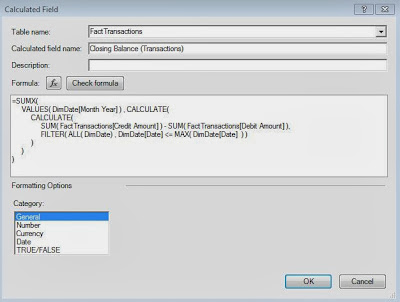Closing Balance (Transactions)
Needless to say, this is not a beginner's calculation.  It requires knowledge of filter context and row context, as well as how context propagates across calculates.  At the heart of this calculation is the idea that we need add up all the credits for a month and subtract all the debits for a month.  The rest is just syntax.  Now, let's see if they add up.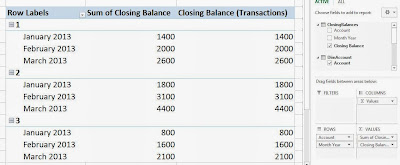Closing Balances by Account and Month Year (Power Pivot)
As you can see, the numbers match perfectly and your boss is now very happy.  Let's see how Tableau would handle this.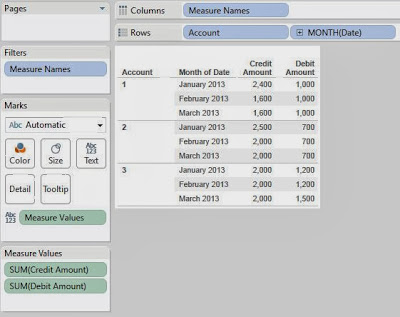Credit Amount and Debit Amount by Account and Month Year (Tableau)
As always with Tableau, we need to create our canvas first.  In this case, we want to use Account by Month, just like in Power Pivot.  Now, we need to create our calculation.Running Sum of Credit (Tableau)
This calculation is probably the simplest of all table calculations.  All we need to do is add up the credits for this month and all previous months, aka a running sum.Running Sum of Credit by Account and Month Year (Tableau)
The only caveat here is that we need to set the "Compute Using" to Pane.  This allows the calculation to reset for each Account.  Next, we can repeat this process for the debits.Balance (Tableau)
Finally, we calculate our balance by subtracting our two running sums.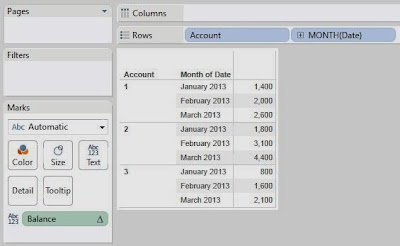Balance by Account and Month Year (Tableau)
As you can see, these are the same values we got in Power Pivot.  Now, we need to add Closing Balance to this sheet.Balance and Closing Balance by Account and Month Year (Incorrect) (Tableau)
As you can see, the closing balances are blending properly across Account (which exists in both tables) but not across MY(Date) (which doesn't exist in the closing balance table).  So, we need to tell Tableau that it can blend MY(Date) with Month Year.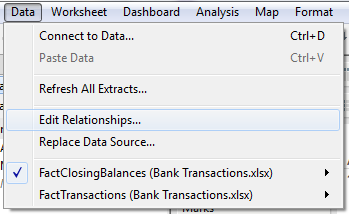Edit Relationships (Tableau)
In the data menu, we can go to "Edit Relationships."  This menu will allow us to tell Tableau how to blend across data sources.Blend MY with Month Year (Tableau)
Next, we need to tell Tableau that it can blend MY(Date) with Month Year.Balance and Closing Balance by Account and Month Year (Correct) (Tableau)
Finally, we need to make sure that the connections are being used.  We can tell by looking at the Orange chain links in the Dimensions Shelf.  We can see that the balances are in fact the same and we have kept our job.

Completing this task in Power Pivot took an advanced knowledge of DAX calculations.  A beginner would have significant trouble with accomplishing this.  In fact, we had so much trouble with it that we had to consult our colleague for assistance.  Tableau, on the other hand, took only a beginner's knowledge of table calculations and how to edit a relationship.  Any intermediate user should be able to complete this task without much trouble.  Therefore, we can make our decision with ease.

Winner: Tableau

Many thanks to our colleague, Javier Guillen, for helping us with the Power Pivot portion of this model.

Model 2: Many-to-Many (M2M) Relationship

The M2M relationship is one of the more complex modeling scenarios you will find.  It seems to pop up in almost every scenario and each case is different.  We will limit this model to a very simple M2M scenario.  We have closing balances for 3 accounts.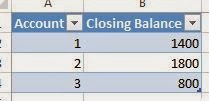Closing Balances (Many-to-Many Relationship)
We also have a list of customers linked to each account.Customers by Account
As you can see, some of these accounts are joint accounts that have multiple customers linked to them.  To make it even worse, John has 2 accounts, one of each type.  So, why is this a problem?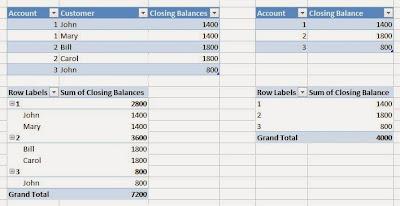Naive Many-to-Many Relationship
As you can see, if you naively join these tables, the totals will be off because some of the balances will be duplicated.  This is the epitome of the M2M relationship.  So, how would you fix these totals?  Let's try it in Power Pivot first.Many-to-Many Relationship (Power Pivot)
The most important part of any Power Pivot analysis is the model.  In this case, we can see that the Customer dimension isn't even connected to the fact table.  This is the issue we have to overcome.  A very interesting blog post was written on this topic and can be found here.  Let's see the resulting calculation.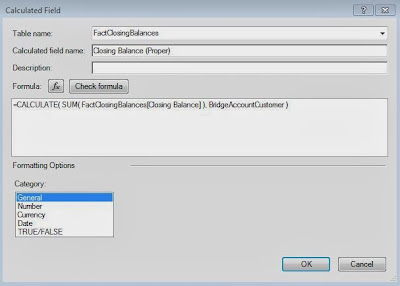Closing Balance (Proper) (Power Pivot)
As you can see, this calculation is simply 1 line of code.  You wrap your aggregation in a CALCULATE() statement and tell it to use the bridge table.  Power Pivot magically takes care of the rest.  Well, it's not magic, but it sure seems like it the first time you see it.  Let's see how our chart turned out.Closing Balance by Account and Customer (Power Pivot)
All of the totals add up correctly, now we can do our analysis on this data without having to worry about duplicates.  Power Pivot even gives us an error letting us know that we have tricked it into doing our bidding.  Let's move on to Tableau.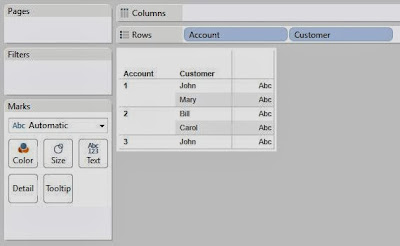Customers by Account (Tableau)
We start by pulling Account and Customer from the bridge table.Closing Balance by Account and Customer (Tableau)
Then, we simply drag Closing Balance onto the chart and the blending takes care of the rest.  Frankly, we work with Tableau every day and were still amazed that blending handled this situation so easily.  This one was an easy decision.

Winner: Tableau

Summary

First, we saw that rolling up account balances is not a trivial task in either of these tools.  However, Tableau showed us how it's blending mechanic can help out in these situations.  Then, we saw that Power Pivot and Tableau were very strong at resolving Many-to-Many relationship.  However, Tableau edged out Power Pivot because of the simplicity of data blending.  We hope you found this informative.  Thanks for reading.

P.S.

This is the last post in the beginning part of this series.  From now on, the posts will focus on specific, advanced situations.  We also will not be able to keep up the weekly pace that we have been.  If you have any specific scenarios you'd like to see us test, send us an email and we'll see what we can do.